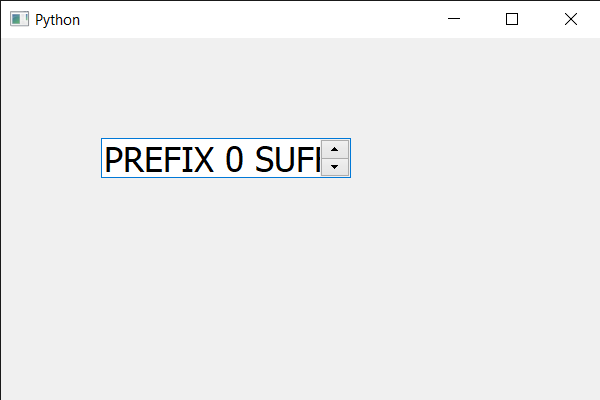GeeksforGeeks App
Open AppBrowser
Continue

# PyQt5 QSpinBox – Setting float point size | setPointSizeF() method

In this article we will see how we can set the floating-point size of the text in spin box. Changing the point size will change the size of the text, pixel and point are two different things some point can have multiple pixels inside it. It is recommended to change point size for changing the size of the text. Unlike normal point size floating-point size can make the point size in decimal as point size is not a pixel its value can be in float as well. In order to do this we use setPointSizeF method with the QFont object of the spin box.

Syntax : font.setPointSizeF(n) Argument : It takes float as argument Return : It returns None

Below is the implementation

## Python3

 `# importing libraries``from` `PyQt5.QtWidgets ``import` `*``from` `PyQt5 ``import` `QtCore, QtGui``from` `PyQt5.QtGui ``import` `*``from` `PyQt5.QtCore ``import` `*``import` `sys`  `class` `Window(QMainWindow):` `    ``def` `__init__(``self``):``        ``super``().__init__()` `        ``# setting title``        ``self``.setWindowTitle("Python ")` `        ``# setting geometry``        ``self``.setGeometry(``100``, ``100``, ``600``, ``400``)` `        ``# calling method``        ``self``.UiComponents()` `        ``# showing all the widgets``        ``self``.show()` `        ``# method for widgets``    ``def` `UiComponents(``self``):``        ``# creating spin box``        ``self``.spin ``=` `QSpinBox(``self``)` `        ``# setting geometry to spin box``        ``self``.spin.setGeometry(``100``, ``100``, ``250``, ``40``)` `        ``# setting range to the spin box``        ``self``.spin.setRange(``0``, ``999999``)` `        ``# setting prefix to spin``        ``self``.spin.setPrefix("PREFIX ")` `        ``# setting suffix to spin``        ``self``.spin.setSuffix(" SUFFIX")` `        ``# getting font of the spin box``        ``font ``=` `self``.spin.font()` `        ``# setting point size F``        ``font.setPointSizeF(``20.25``)` `        ``# reassigning this font to the spin box``        ``self``.spin.setFont(font)` `       `  `# create pyqt5 app``App ``=` `QApplication(sys.argv)` `# create the instance of our Window``window ``=` `Window()` `# start the app``sys.exit(App.``exec``())`

Output :My Personal Notes arrow_drop_up